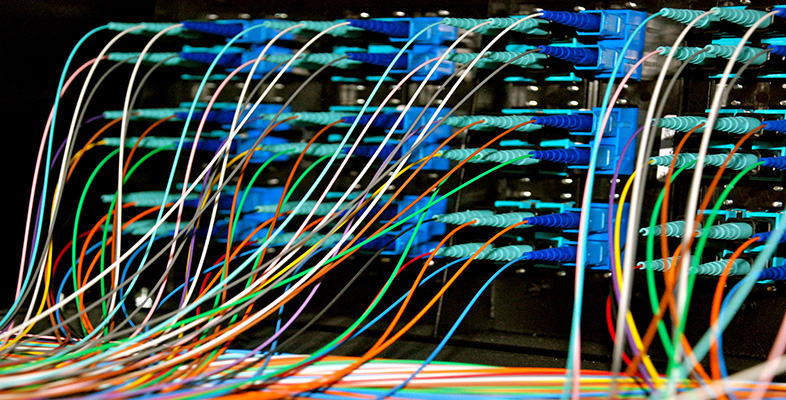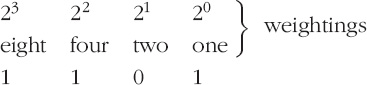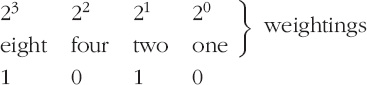Representing and manipulating data in computers

This free course is available to start right now. Review the full course description and key learning outcomes and create an account and enrol if you want a free statement of participation.

Free course

# 2.2.2 Positive integers: binary numbers

Just as a denary number system uses ten different digits (0, 1, 2, 3, … 9), a binary number system uses two (0, 1).

Once again the idea of positional notation is important. You have just seen that the weightings which apply to the digits in a denary number are the exponents of ten. With binary numbers, where only two digits are used, the weightings applied to the digits are exponents of two.

The rightmost bit is given the weighting of 2°, which is 1. The next bit to the left is given a weighting of 21, which is 2, and so on.

Thus the 4-bit binary number 1101 represents:The leftmost bit is called the most-significant bit (m.s.b.) and the rightmost bit is called the least-significant bit (l.s.b.)

Computers are designed to work with binary numbers, but denary numbers suit most people better. Humans therefore need to interpret the 4-bit binary number 1101 as follows:

(1 × 23) + (1 × 22) + (0 × 21) + (1 × 20)

which is 8 + 4 + 0+ 1 = 13 in denary.

## Activity 3 (Self assessment)

What does the 4-bit binary number 1010 represent? To what denary number is it equal? What is its most-significant bit?

1010 representsIt is equal to 8 + 0 + 2 + 0 = 10 in denary and its most-significant bit is 1.

Exactly the same principles can be applied to larger binary numbers. For example, in an 8-bit word the most-significant bit has a weighting of 27, which is 128. So 10110110 represents:

and equals 128 + 0 + 32 + 16 + 0+ 4 + 2 + 0, which is 182 in denary.

It is often convenient to number the bits in a binary word and to refer to the bits as ‘bit 5’ or ‘bit 2’. And the most convenient way of numbering the bits is to use the exponents in the weightings. So the bits are usually numbered as follows (for an 8-bit word):

 7 6 5 4 3 2 1 0 bit number 27 26 25 24 23 22 21 20 weighting

This scheme means that the least-significant bit is bit 0 and the most-significant bit is bit 7.

Just as a space between groups of three digits in large numbers (e.g. 625 127) can make them easier to read, so spaces between groups of four bits can make binary numbers easier to read. I shall use spaces in this way. So I shall write, for example, 1001 1110 rather than 10011110. Note that the space is purely for convenience in reading the number; it does not affect the value of the number in any way, nor the way the computer handles it.

## Activity 4 (Self assessment)

1. To what denary number is the binary number 1100 0110 equal?

2. In a 16-bit word, what is the weighting of the most-significant bit? What bit number should be allocated to this bit?

3. To what denary number is the binary number 1000 0000 0000 0001 equal?

Note that Windows has a calculator (in Accessories) that will evaluate numbers like 210, 214, etc. You will need to choose Scientific from its View menu and then use the x^y key. For instance, to find 210 press 2 then x^y then 10 then =. You should get 1024.

1. Omitting the zeros because they do not contribute to the sum, this binary number is equal to:

(1 × 27) + (1 × 26) + (1 × 22) + (1 × 21)

which is 128 + 64 + 4+ 2 = 198 in denary.

2. In an 8-bit word the weighting of the most-significant bit is 27. By noting that 7 is one less than 8 you can see that the weighting of the most-significant bit in a 16-bit word will be 215. 215 is equal to 32 768 and the bit number is 15 (it is the same as the exponent of 2).

3. It is equal to:

(1 × 215) + (1 × 20)

which is 32 768 + 1 = 32 769 in denary.

Counting in binary is very simple because there are only two possible digits. It proceeds as follows:

• 0

• 1

• 10

• 11

• 100

• 101

• 110

• 111

• 1000

• etc.

Notice that when all the bits in any number are 1, the next higher number needs one more bit to represent it. This is exactly analogous to denary, where 999 is followed by 1000, which needs one more digit to represent it.

## Activity 5 (Self assessment)

• (a) What binary number follows

• (i) 1 1111

• (ii) 1 0111?

• (b) What binary number precedes 1000 0000?

• (a) (i)10 0000

• (a) (ii) 1 1000

(b) 111 1111 (or 0111 1111 if an 8-bit word is to be used).

T224_2